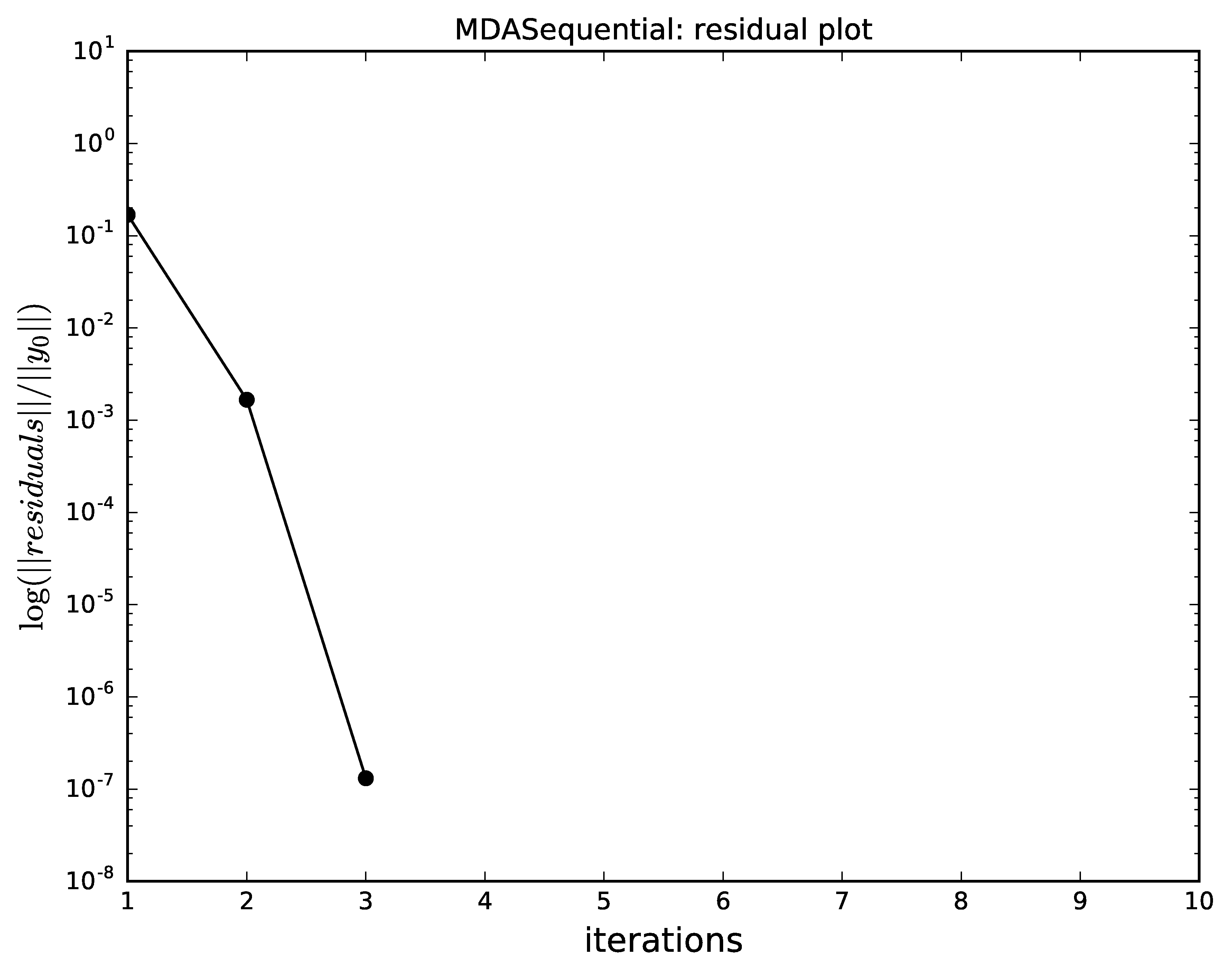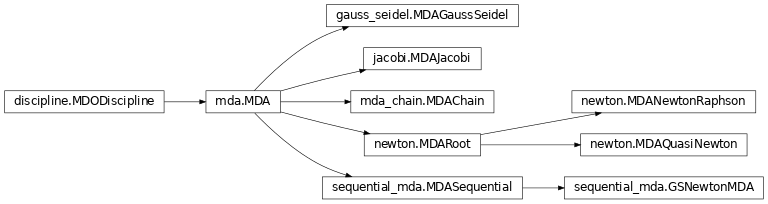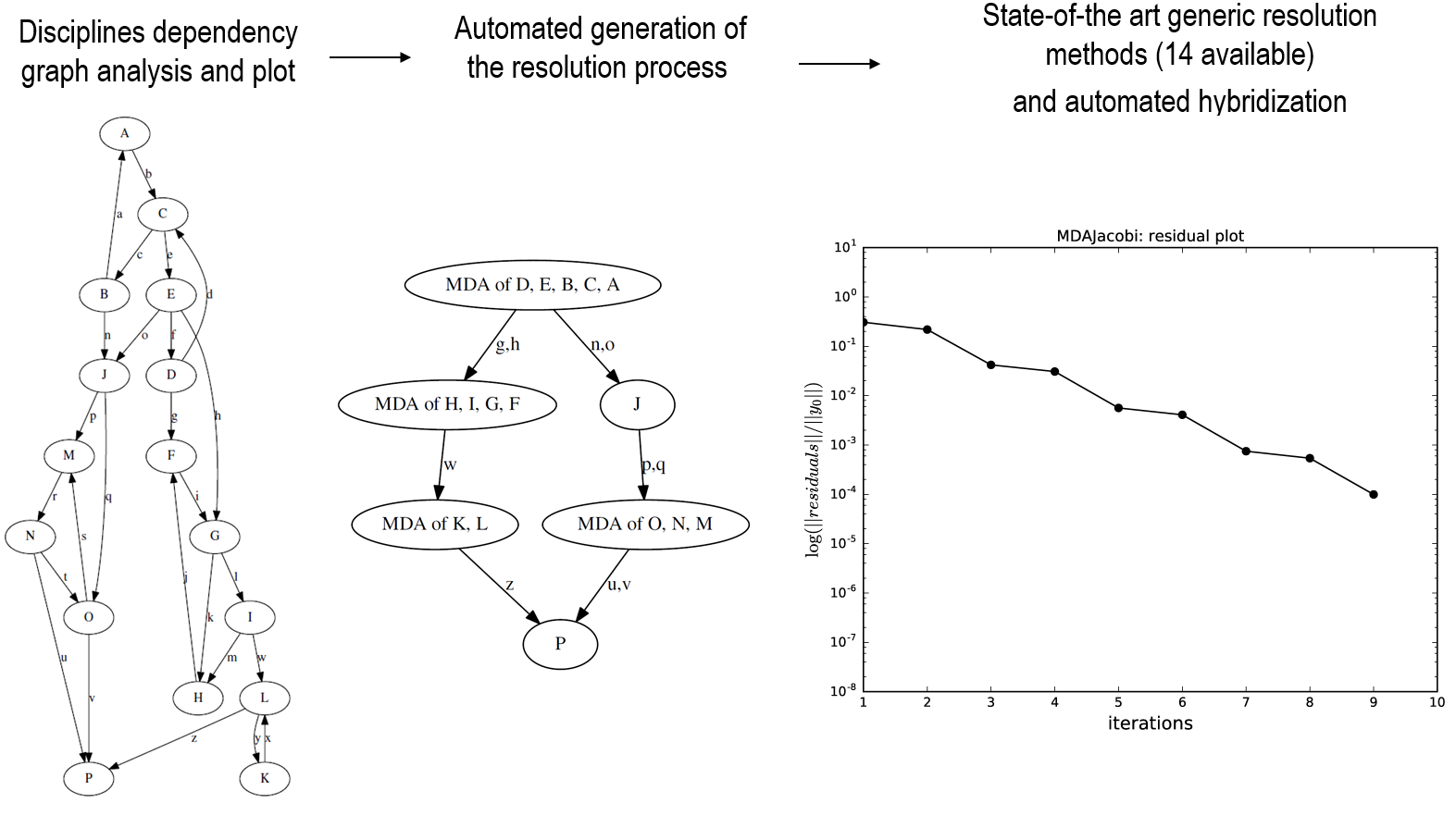# Multi Disciplinary Analyses¶

This section is concerned with the construction and execution of a Multi Disciplinary Analysis (MDA), i.e. the computation and the convergence of the coupling variables of coupled disciplines.

## Creation of the MDAs¶

Two families of MDA methods are implemented in GEMSEO and can be instanciated through the API function create_mda():

• fixed point algorithms, that compute fixed points of a coupling function $$y$$, that is they solve the system $$y(x) = x$$

• Newton method algorithms for root finding, that solve a non-linear multivariate problem $$R(x, y) = 0$$ using the derivatives $$\frac{\partial R(x, y)}{\partial y} = 0$$ where $$R(x, y)$$ is the residual.

Any explicit problem of the form $$y(x) = x$$ can be reformulated into a root finding problem by stating $$R(x, y) = y(x) - x =0$$. The opposite is not true. This means that if the disciplines are provided in a residual form, the Gauss-Seidel algorithm for instance cannot be directly used.

### Fixed-point algorithms¶

Fixed-point algorithms include Gauss-Seidel algorithm and Jacobi algorithms, each of which is implemented by a class:

from gemseo.api import create_mda, create_discipline

disciplines = create_discipline(["SobieskiPropulsion", "SobieskiAerodynamics",
"SobieskiMission", "SobieskiStructure"])

mda_gaussseidel = create_mda("MDAGaussSeidel", disciplines)
mda_jacobi = create_mda("MDAJacobi", disciplines)


The classes MDAGaussSeidel and MDAJacobi called by create_mda() inherit from MDA, itself inheriting from MDODiscipline. Therefore, an MDA based on a fixed-point algorithm can be viewed as a discipline whose inputs are design variables and outputs are coupling variables.

### Root finding methods¶

Root finding methods include Newton-Raphson and quasi-Newton methods, each of which is implemented by a class.

#### Newton-Raphson method¶

The Newton-Raphson method is parameterized by a relaxation factor $$\alpha \in (0, 1]$$ to limit the length of the steps taken along the Newton direction. The new iterate is given by: $$x_{k+1} = x_k - \alpha f'(x_k)^{-1} f(x_k)$$.

mda = create_mda("MDANewtonRaphson", disciplines, relax_factor=0.99)


#### Quasi-Newton method¶

Quasi-Newton methods include numerous variants (Broyden, Levenberg-Marquardt, …). The name of the variant should be provided as a parameter method of the class.

mda = create_mda("MDAQuasiNewton", disciplines, method=MDAQuasiNewton.BROYDEN1)


The classes MDANewtonRaphson and MDAQuasiNewton called by create_mda() inherit from MDARoot, itself inheriting from MDA, itself inheriting from MDODiscipline. Therefore, an MDA based on a root finding method can be viewed as a discipline whose inputs are design variables and outputs are coupling variables.

### Hybrid methods¶

Hybrid methods implement a generic scheme to hybridize elementary MDAs: an arbitrary number of them are provided at instantiation of the class, and they are executed sequentially. The following code creates a hybrid mda that runs sequentially one iteration of Jacobi method mda1 and a full Newton-Raphson method mda2.

mda1 = create_mda("MDAJacobi", disciplines, max_mda_iter=1)
mda2 = create_mda("MDANewtonRaphson", disciplines)
mda = create_mda("MDASequential", disciplines, mda_sequence = [mda1, mda2])


This sequence is typically used to take advantage of the robustness of fixed-point methods and then obtain accurate results thanks to Newton methods.

## Execution and convergence analysis¶

The MDAs are run using the default input data of the disciplines as a starting point. The MDA provides a method that plots the evolution of the residuals of the system with the iterations; the plot may be displayed and/or saved to a PDF file by means of the function plot_residual_history():

mda.plot_residual_history(n_iterations=10, logscale=[1e-8, 10.])


The next plots compare the convergence of Gauss-Seidel, Jacobi, quasi-Newton and the hybrid with respect to the iterations. Identical scales were used for the plots (n_iterations for the $$x$$ axis and logscale for the logarithmic $$y$$ axis, respectively). It shows that, as expected, Gauss-Seidel has a better convergence than the Jacobi method. The hybrid MDA, combining an iteration of Jacobi and a full Newton-Raphson, converges must faster than all the other alternatives; note that Newton-Raphson alone does not converge for this initial values of the coupling variables.Hybrid Gauss-Seidel and Quasi-Newton algorithm convergence for MDA

## Classes organization¶

The following inheritance diagram shows the different MDA classes in GEMSEO and their organization.## MDAChain and the Coupling structure for smart MDAs¶

The MDOCouplingStructure class encompasses the coupled and provides methods to compute the coupling variables between the disciplines:

from gemseo.core.coupling_structure import MDOCouplingStructure

coupling_structure = MDOCouplingStructure(disciplines)


This is an internal object that is created in all MDA classes and all formulations. The user does not need to create it for basic usage, he creates MDAs instead.

The MDOCouplingStructure uses graphs to compute the dependencies between the disciplines, and therefore the coupling variables. This graph can then be used to generate a process to solve the coupling problem with a coupling algorithm.

To illustrate the typical procedure, we take a dummy 16 disciplines problem.

1. First the coupling graph is generated.

2. Then, a minimal process is computed, with eventually sub MDAs. A set of coupling problems is generated, which are passed to algorithms.

3. Finally, many zero search solvers algorithms are available in GEMSEO (14), via the SciPy package, or directly coded in GEMSEO (Gauss-Seidel and Jacobi for instance). They can be compared on the specific problem, and MDAs can generate convergence plots of the residuals.

The next figure illustrates this typical processThe 3 resolution phases of a 16 disciplines coupling problem

This features is used in the MDAChain which generates a chain of MDAs according to the graph of dependency in order to minimize the CPU time. The user provides a base MDA class to solve the coupled problems. The overall sequential process made of sub-MDAs and disciplines execution is created by a MDOChain. The sub-MDAs can be specified using the argument sub_mda_class.

mda = create_mda("MDAChain", disciplines, sub_mda_class="MDAJacobi")
mda.execute()